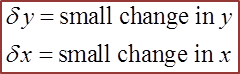# 9.9 Small Changes and Approximations

9.9 Small Changes and Approximations,This is very useful information in determining an approximation of the change in one variable given the small change in the second variable.

Example:
Given that y = 3x2 + 2x – 4. Use differentiation to find the small change in y when x increases from 2 to 2.02.

Solution:
$\begin{array}{l}y=3{x}^{2}+2x-4\\ \frac{dy}{dx}=6x+2\end{array}$

The small change in is denoted by δy while the small change in the second quantity that can be seen in the question is the x and is denoted by δx.